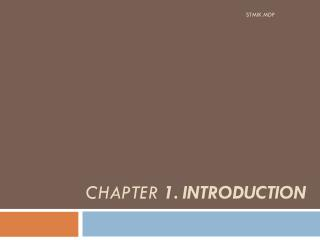# CHAPTER 1. INTRODUCTION - PowerPoint PPT PresentationDownload PresentationCHAPTER 1. INTRODUCTION

CHAPTER 1. INTRODUCTIONDownload Presentation## CHAPTER 1. INTRODUCTION

- - - - - - - - - - - - - - - - - - - - - - - - - - - E N D - - - - - - - - - - - - - - - - - - - - - - - - - - -
##### Presentation Transcript

1. STMIK MDP CHAPTER 1. INTRODUCTION

2. Figure 1.1 : A compiler STMIK MDP

3. Figure 1.3: An interpreter STMIK MDP

4. Figure 1.4: A hybrid compiler STMIK MDP

5. Figure 1.6: Phases of a compiler STMIK MDP

6. Figure 1.10: Blocks in a C++ program STMIK MDP

7. Figure 1.13: Block-structured code STMIK MDP

8. Next A Simple Syntax – Directed Translator STMIK MDP